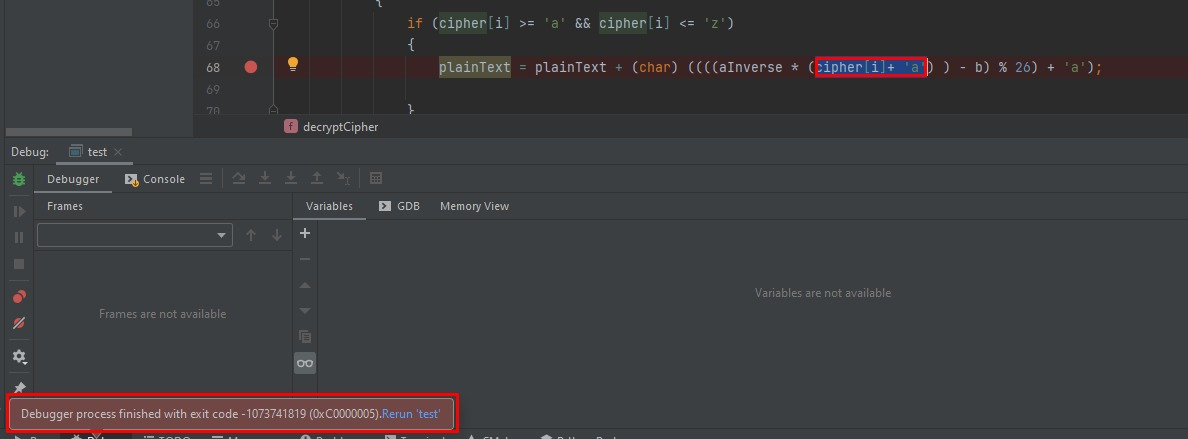The debugger keeps stopping. In any C++ program I try to debug, I get this annoying message (Debugger process finished with exit code -1073741819 (0xC0000005)), which right after it, the debugger stops working and I have to rerun it again.

For example when I try to highlight that portion of the code - to evaluate it. After pressing right key I get the red pop-up message of the debugger stopped working.and this is the code I was debugging.

<code>

`#include<bits/stdc++.h>using namespace std;//Key values of a and bconst int a = 17;const int b = 20;string encryptMessage(string plainText){    string cipher = "";    for (int i = 0; i < plainText.length(); i++)    {        if(plainText[i]!=' ')        {            if ((plainText[i] >= 'a' && plainText[i] <= 'z') || (plainText[i] >= 'A' && plainText[i] <= 'Z'))            {                if (plainText[i] >= 'a' && plainText[i] <= 'z')                {                    cipher = cipher + (char) ((((a * (plainText[i]-'a') ) + b) % 26) + 'a');                }                else if (plainText[i] >= 'A' && plainText[i] <= 'Z')                {                    cipher = cipher + (char) ((((a * (plainText[i]-'A') ) + b) % 26) + 'A');                }            }            else            {                cipher += plainText[i];            }        }        else        {            cipher += plainText[i];        }    }    return cipher;}string decryptCipher(string cipher){    string plainText = "";    int aInverse = 0;    int flag = 0;    for (int i = 0; i < 26; i++)    {        flag = (a * i) % 26;        if (flag == 1)        {            aInverse = i;        }    }    for (int i = 0; i < cipher.length(); i++)    {        if(cipher[i] != ' ')        {            if ((cipher[i] >= 'a' && cipher[i] <= 'z') || (cipher[i] >= 'A' && cipher[i] <= 'Z'))            {                if (cipher[i] >= 'a' && cipher[i] <= 'z')                {                    plainText = plainText + (char) ((((aInverse * (cipher[i]+ 'a') ) - b) % 26) + 'a');                }                else if (cipher[i] >= 'A' && cipher[i] <= 'Z')                {                    plainText = plainText + (char) (((aInverse * ((cipher[i]+'A' - b)) % 26)) + 'A');                }            }            else            {                plainText += cipher[i];            }        }        else            plainText += cipher[i];    }    return plainText;}//Driver Programint main(void){    string msg = "abcdefghijklmnopqrstuvwxyz ABCDEFGHIJKLMNOPQRSTUVWXYZ";    //Calling encryption function    string cipherText = encryptMessage(msg);    cout << "Encrypted Message is : " << cipherText<<endl;    //Calling Decryption function    cout << "Decrypted Message is : " << decryptCipher(cipherText);    return 0;}`

</code>

1 commentHello!

It seems to be a bug inside GDB itself. The solution is either to update the MinGW-W64 package for GDB, or to use another toolchain.